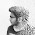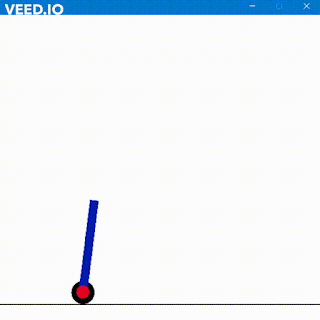## VEDIC MULTIPLIER

Hola Amigos
Multiplication in verilog seems an easy task however one must use and appropriate algorithm to save memory, space, RTL complexity, and performance.
You all will find certainly various multipliers including the famous booth multiplier. I followed a different algo since I was having a tough time to understand Booth algo. My multiplication follows the methedology of Vedic Multiplier. Many of my amigos must have heard of it.

Let me explain.
Suppose we have two 8 bit numbers as 16 and 16. Multiplying them would double up the bits as per conventional mathematics thus the result will be 256 in 16 bits.

Hence we will have input as
input [7:0] a,b;

and output as
output reg [7:0]c;

Output can also be a wire but then you will have to use assign keyword. It won't remain under initial block or always block. Hence I have taken it as reg type to be inside always block.

The basic module or better say fundamental module starts with 2bit vedic multiplier.
Taking 2bit case

a = 10 and b = 10 then
Step 1- AND of a with b
Step 2 - Cross AND of a with b
Step 3- Cross AND of a with b
Step 4- Cross AND of b with a
Step 5  - ADD them side by side

Here is the blessing!

Thus similarly for 4bit vedic multiplierif a = 1010 and b = 1101
Then we will have to perform
Step 1 - 2bit vedic for a[3:2] and b[3:2] then concat 00 after result totaling 6bits
-----------
Step 2 - 2bit vedic for a[1:0] and b[3:2] then concat 00 before result  totaling 6 bits
------------
Step 3 - add result of step1 and step2
------------
Step 4 - 2bit vedic for a[3:2] and b[1:0]
------------
Step 5 - 2bit vedic for a[1:0] and b[1:0]
------------
Step 6 - Let result of step6 be q then concat 00 before q[3:2]
------------
Step 7 - add result of step 6 and step 5
------------
Step 9 - add result of step 7 and step 3
The result is x[7:2]
and x[1:0] will be straight q[1:0]
Concating both we have c[7:0] result

Similarly here is the

Code for 8bit Multiplier

module Eight_vedic(a,b,c);
input [7:0] a;
input [7:0] b;
output [15:0] c;
wire [31:0] wir;
wire [11:0] wir2;
wire [11:0] wir3;
wire [11:0] result1;
wire [11:0] result2;
wire wir6;
//assign c = 15'b0;
Four_vedic v1(a[7:4],b[7:4],wir[31:24]);
Four_vedic v2(a[3:0],b[7:4],wir[23:16]);
assign wir2 = {wir[31:24],4'b0};
assign wir3 = {4'b0,wir[23:16]};
assign result1 = wir2 + wir3;
Four_vedic v3(a[7:4],b[3:0],wir[15:8]);
Four_vedic v4(a[3:0],b[3:0],wir[7:0]);
assign result2 = result1 + {4'b0,wir[15:8]}+{8'b0,wir[7:4]};
assign c[3:0] = wir[3:0];
assign c[15:4] = result2[11:0];

endmodule

Code for 4bit vedic

module Four_vedic(a,b,c);
input [3:0] a;
input [3:0] b;
output [7:0] c;
wire [15:0] wir;
wire [5:0] wir2;
wire [5:0] wir3;
wire [5:0] result1;
wire [5:0] result2;
wire wir6;
Two_vedic v1(a[3:2],b[3:2],wir[15:12]);
Two_vedic v2(a[1:0],b[3:2],wir[11:8]);
assign wir2 = {wir[15:12],2'b0};
assign wir3 = {2'b0,wir[11:8]};
assign result1 = wir2 + wir3;
Two_vedic v3(a[3:2],b[1:0],wir[7:4]);
Two_vedic v4(a[1:0],b[1:0],wir[3:0]);
assign result2 = result1 + {2'b0,wir[7:4]}+{4'b0,wir[3:2]};
assign c[1:0] = wir[1:0];
assign c[7:2] = result2[5:0];
endmodule

Code for 2bit vedic
module Two_vedic(a,b,c);
input [1:0] a;
input [1:0] b;
output [3:0] c;
wire [3:0] wir;
assign wir = a & b;
assign wir = (a&b)^(a&b);
assign wir = (a&b)&(a&b)^(a&b);
assign wir = (a&b)&(a&b)&(a&b);
assign c = {wir,wir,wir,wir};
endmodule

Testbench
module test();
reg [7:0]a;
reg [7:0]b;
wire [15:0]s;
initial begin
a = 5;
b = 4;
#100 a = 7;
b = 3;
#100 a = 9;
b = 8;
#100 a = 4;
b = 4;
#100 a = 256;
b = 256;
#100 a = 100;
b = 15;
end
Eight_vedic v(a,b,s);
endmodule

Remember for 8bit vedic both 4 and 2bit modules must be present in code.
Similarly for 16bit all 8 and 4 and 2 bit will remain in module.
16 bit testbench will call 16bit module which instantiate 8bit which will instantiate 4bit and in continuum 2bit will be instantiated. One cannot skip any module.

EDIT- 16bit vedic will be released with patch 1.0.0

So Long

1.can you ,please post the explanation for this code in detail?

1.Will surely do so if I get some time.

2.Suman can you please check the procedure of 2x2 bit vedic multiplier steps in above.

2.i want to 32 bit vedic multiplier coding....

3.Sir please send 16 bit code

### Self Balancing Robot using Machine Learning

Hola Amigos, I have always loved inverted pendulums. They are very fascinating to me and I play with them a lot. In real life, I have m...